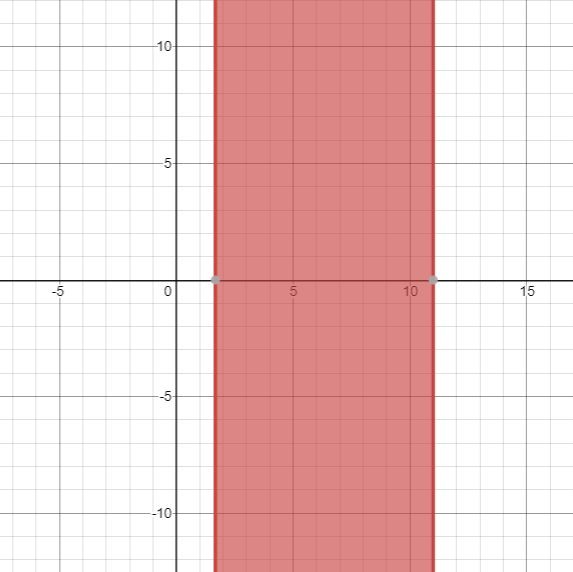# The solution of given linear inequality equation and plot a graph for it.### Precalculus: Mathematics for Calcu...

6th Edition
Stewart + 5 others
Publisher: Cengage Learning
ISBN: 9780840068071### Precalculus: Mathematics for Calcu...

6th Edition
Stewart + 5 others
Publisher: Cengage Learning
ISBN: 9780840068071

#### Solutions

Chapter 1.7, Problem 34E
To determine

## The solution of given linear inequality equation and plot a graph for it.

Expert Solution

1.67x11

### Explanation of Solution

Given:

Linear inequality equation

1243x514

Calculation,

Linear inequality equation,

1243x51410204(43x)20520204(43x)5201612x52012x5162012x112012x112012x111.67x11

Now, graph of 1243x514Conclusion:

Hence, the values of x lies between the 1.67 and 11.

### Have a homework question?

Subscribe to bartleby learn! Ask subject matter experts 30 homework questions each month. Plus, you’ll have access to millions of step-by-step textbook answers!